Related Articles

# Class 8 RD Sharma Solutions – Chapter 27 Introduction to Graphs – Exercise 27.2

• Last Updated : 30 Apr, 2021

### Question 1. The following table shows the number of patients discharged from a hospital with HIV diagnosis in different years:

Represent the information by a graph.

Solution:

Attention reader! All those who say programming isn't for kids, just haven't met the right mentors yet. Join the  Demo Class for First Step to Coding Coursespecifically designed for students of class 8 to 12.

The students will get to learn more about the world of programming in these free classes which will definitely help them in making a wise career choice in the future.

From the question as we know that,

The number of patients – is a dependent variable.

Year – is an independent variable.

Take years on x-axis

and Number of patients on y – axis

Choose the following scale:

X – axis: 2 cm = 1 year

Y – axis: 1 cm = 10 patients

Consider that on the x-axis, origin (O) represents 2001 and on the y-axis, origin (O) represents 120,

i.e. O (2001, 120).

Now, Plot

(2002, 150),

(2003, 170),

(2004, 195),

(2005, 225),

(2006, 230).

Join these points in the following graph as shown below.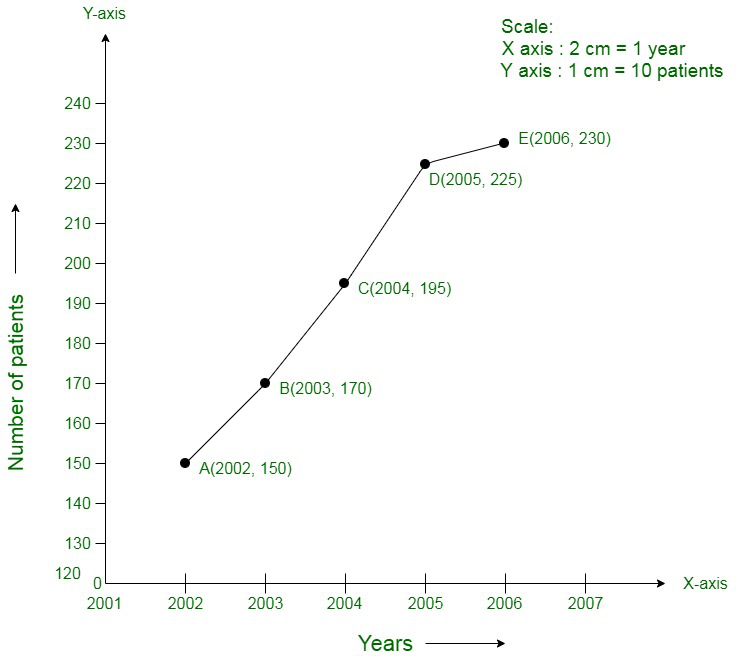### Question 2. The following table shows the amount of rice grown by a farmer in different years:

Plot a graph to illustrate this information.

Solution:

From the question as we know that,

Year – is an independent variable

Quantity of rice grown is a dependent variable.

So, take

Year on X – axis

Quantity of rice grown on Y – axis

Choose following scale:

X – axis: 2 cm = 1 year

Y – axis: 1 cm = 20 quintals

Consider that the origin O represents the coordinates (1999, 160).

i.e. O (1999,160)

Now, Plot

(2000, 200),

(2001, 180),

(2002, 240),

(2003, 260),

(2004, 250),

(2005, 200),

(2006, 270).

Join these points on the following graph as shown below: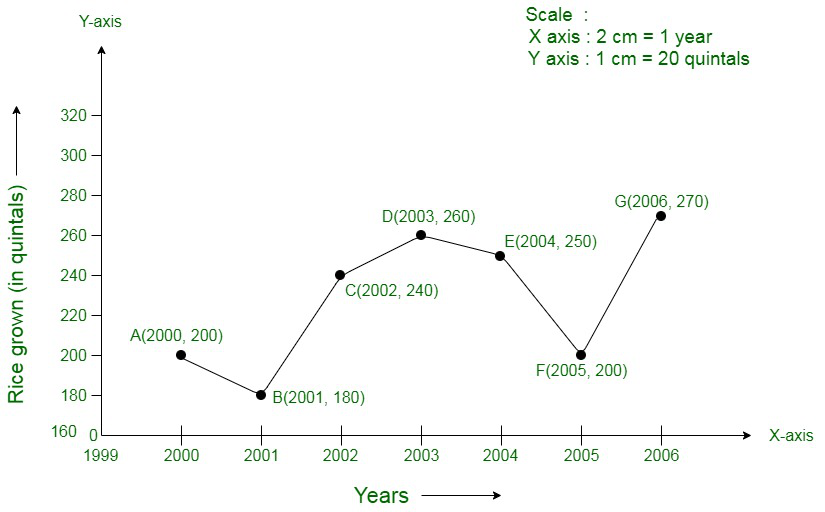### Question 3.The following table gives the information regarding the number of persons employed to a piece of work and time taken to complete the work:

Plot the graph of this information.

Solution:

From the question as we know that,

The number of persons – is an independent variable

Time taken – is a dependent variable.

So, take

The number of persons on the X – axis

Time taken on the Y – axis.

Choose following scale:

X – axis: 2 cm = 2 persons

Y – axis: 2 cm = 2 days

Now, plot

(2, 12),

(4, 6),

(6, 4),

(8, 3).

Join these points on the following graph as shown below: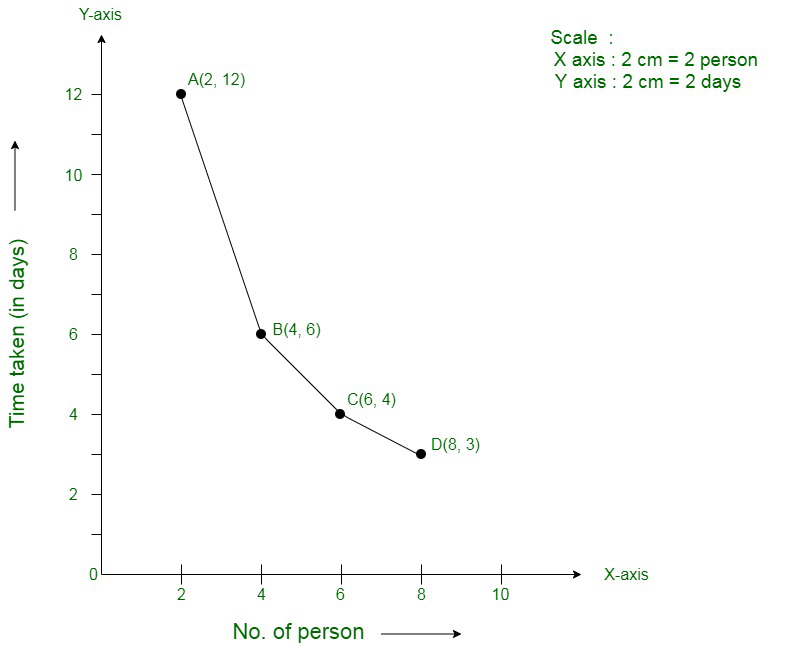### Question 4. The following table gives the information regarding length of a side of a square and its area

Draw a graph to illustrate this information.

Solution:

From the question as we know that,

Length of a side – is an independent variable

Area of the square – is a dependent variable.

So, take

Length of a side on the X – axis

Area of the square on the Y – axis.

Choose following scale:

X – axis: 2 cm = 1 cm

Y – axis: 1 cm = 2 cm2

Now, plot

(1, 1),

(2, 4),

(3, 9),

(4, 16),

(5, 25).

Join these points in the following graph as shown below: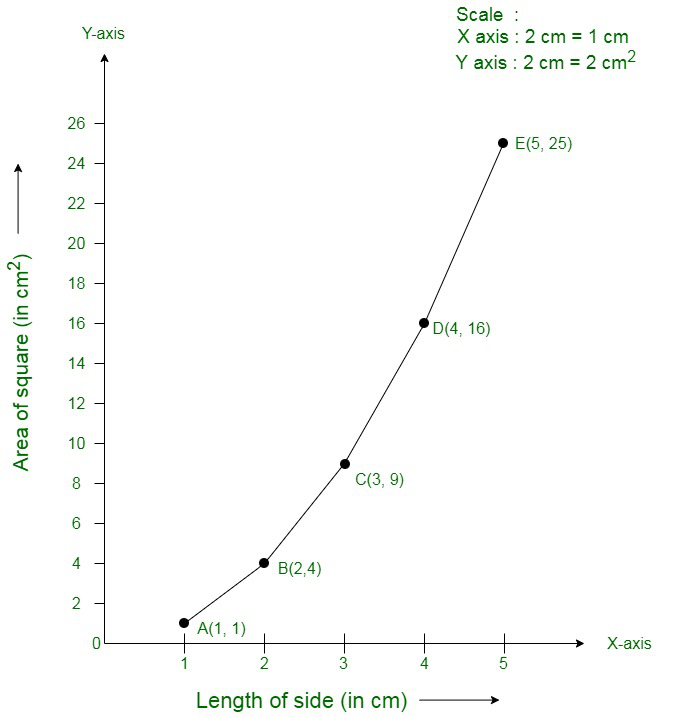### Question 5. The following table shows the sales of a commodity during the years 2000 to 2006.

Draw a graph of this information.

Solution:

From the question as we know that,

Year – is an independent variable

Sales – is a dependent variable.

So, take

Year on the X – axis

Sales on the Y – axis.

Choose following scale:

X – axis: 2 cm = 1 year

Y – axis: 2 cm = 1 lakh rupees

Consider that on x-axis, origin (O) represents 1991.

Hence, the coordinates of O are (1991, 0).

Now, plot

(2000, 1.5),

(2001, 1.8),

(2002, 2.4),

(2003, 3.2),

(2004, 5.4),

(2005, 7.8)

(2006, 8.6).

Join these points on the following graph as shown below;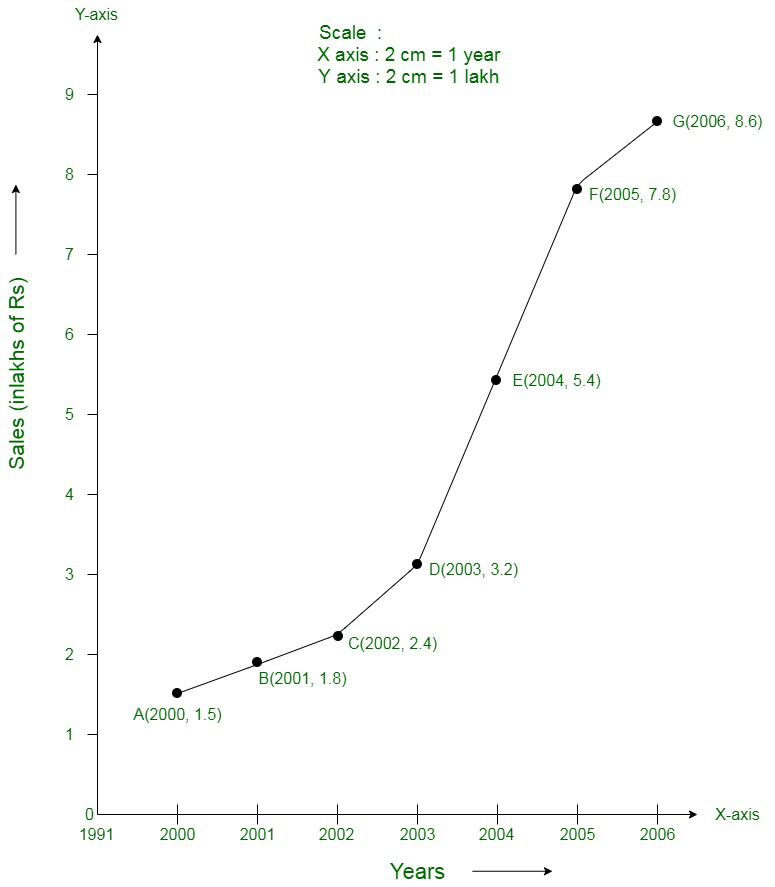### Question 6. Draw the temperature-time graph in each of the following cases:

(i)

(ii)

Solution:

(i) From the question as we know that,

Time – is an independent variable

Temperature – is a dependent variable.

So, take

Time on the X – axis

Temperature on the Y – axis.

Choose following scale:

X – axis: 1 cm = 1 hour

Y – axis: 1 cm = 1°F

Consider that on the x-axis, the coordinate of origin is 6:00.

On y-axis, the coordinate of origin is 94°F.

Hence, the coordinates of 0 are (6:00, 94).

Now, plot

(7:00, 100),

(9:00, 101),

(11:00, 104)

(21:00, 98)

Join these points on the following graph as shown below: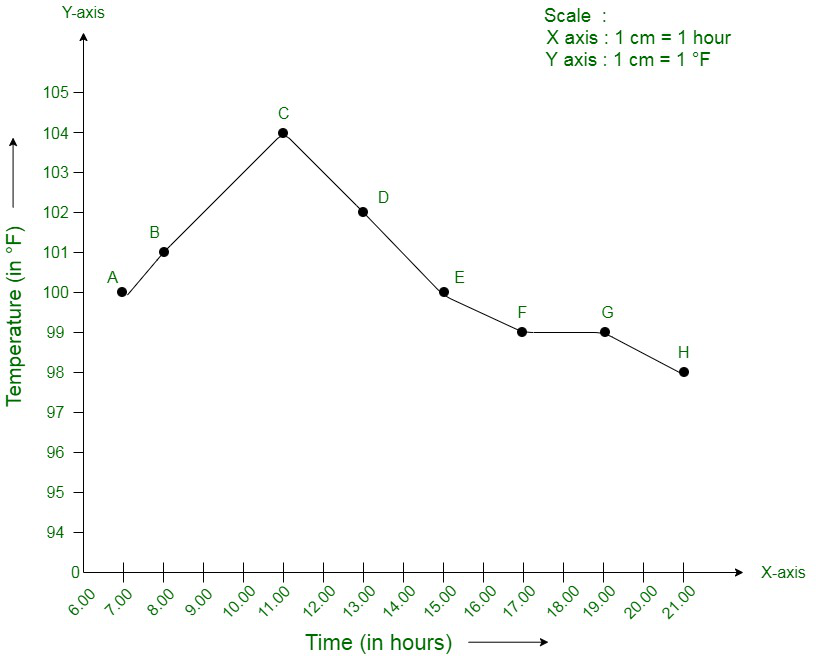(ii) From the question we know that,

Time – is an independent variable

Temperature – is a dependent variable.

So, take

Time on the X – axis

Temperature on the Y – axis.

Choose following scale:

X – axis: 2 cm = 2 hours

Y – axis: 1 cm = 1°F

Consider that on the x-axis, the coordinate of origin is 6:00.

On y-axis, the coordinate of origin is 94°F.

Thus, the coordinates of 0 are (6:00, 94).

Now, plot

(8:00, 100),

(10:00, 101),

(12:00, 104)…..

(20:00, 100).

Join these points on the graph as shown below: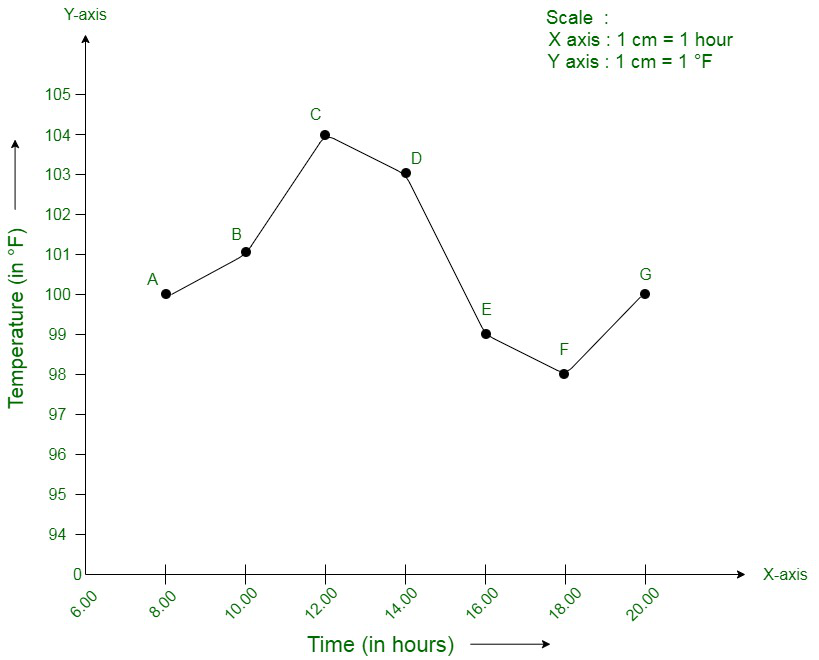### Question 7. Draw the velocity-time graph from the following data:

Solution:

From the question as we know that,

Time – is an independent variable.

Speed – is a dependent variable.

So, take

Time on the X – axis

Speed on the Y – axis.

Choose following scale:

X – axis: 2 big division = 1 hour

Y – axis: 1 big division = 10 km/hr

Consider that on the x-axis, the coordinate of origin (O) is 7:00.

Thus, the coordinates of O are (7:00, 0).

Now, plot

(7:00, 30),

(8:00, 45),

(9:00, 60),

(10:00, 50),

(11:00, 70),

(12:00, 50),

(13:00, 40),

(14:00, 45).

Join these points on the graph as shown below: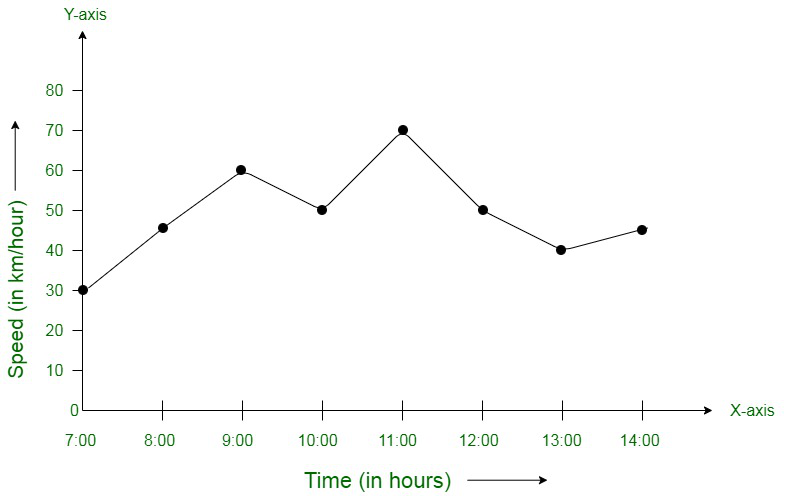### Draw the graph representing the above data in two different ways as a graph and as a bar chart.

Solution:

From the question as we know that,

Over – is an independent variable.

Run is a dependent variable.

So, take

Overs on the X – axis

Runs on the Y – axis.

Choose following scale:

X – axis: 1 cm = 1 over

Y – axis: 1 cm = 2 runs

Now, plot

(I, 2),

(II, 1),

(III, 4)…

(XV, 12).

Join these points on graph as shown below: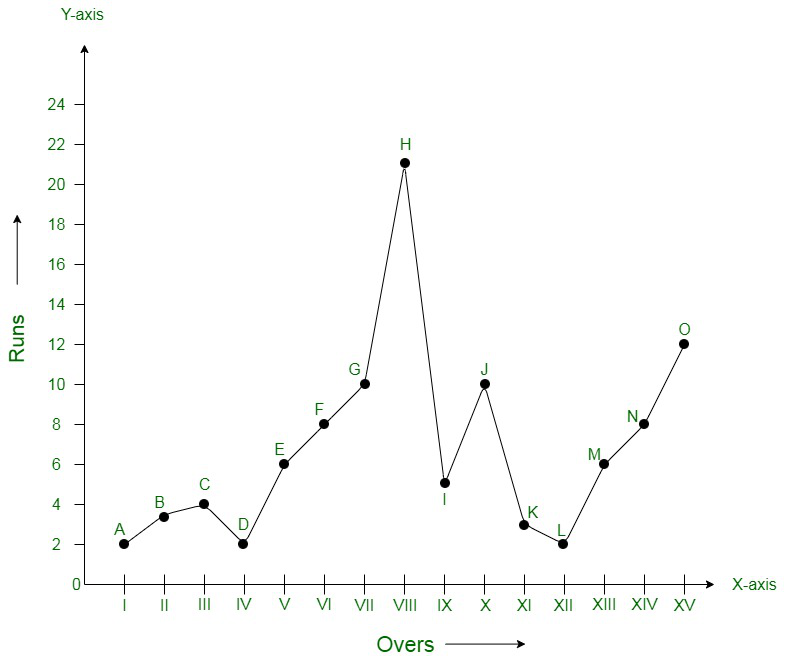The bar graph is: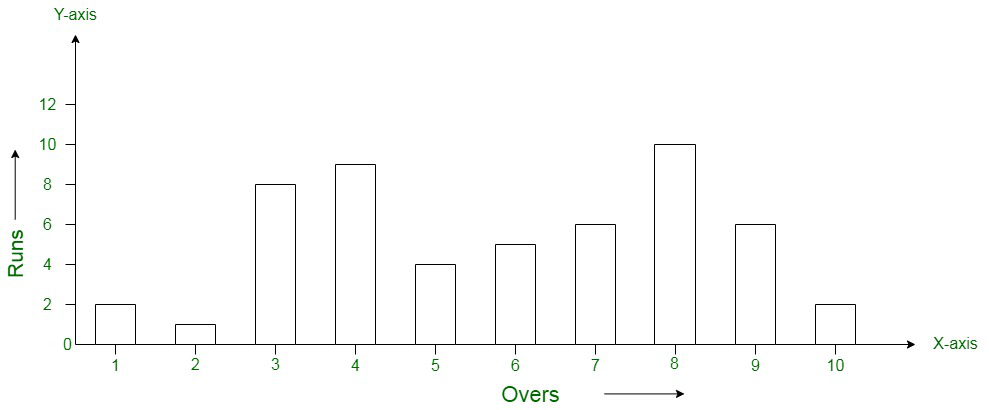### Draw a graph depicting the data, making the graphs on the same axes in each case in two different ways as a graph and as a bar chart.

Solution:

From the question as we know that,

Over – is an independent variable.

Run – is a dependent variable.

So, take

Overs on X – axis

Runs on the Y – axis.

Choose following scale:

X – axis: 1 cm = 1 over

Y – axis: 1 cm = 1 run

Now, plot

(I, 2),

(II, 1),

(III, 8)…

(X, 2) for team A

and

(I, 5),

(II, 6),

(III, 8) …..

(X, 10) for team B.

Join these points on graph as shown below: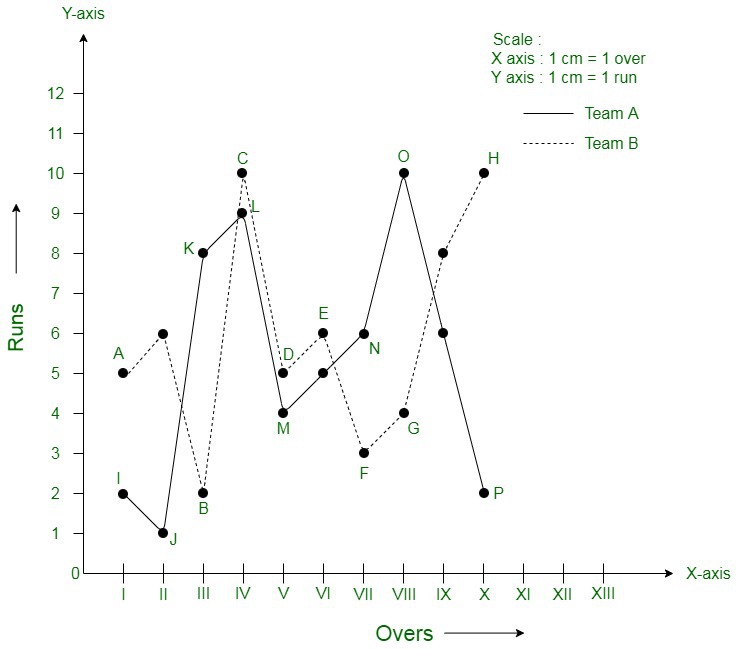The bar graph is: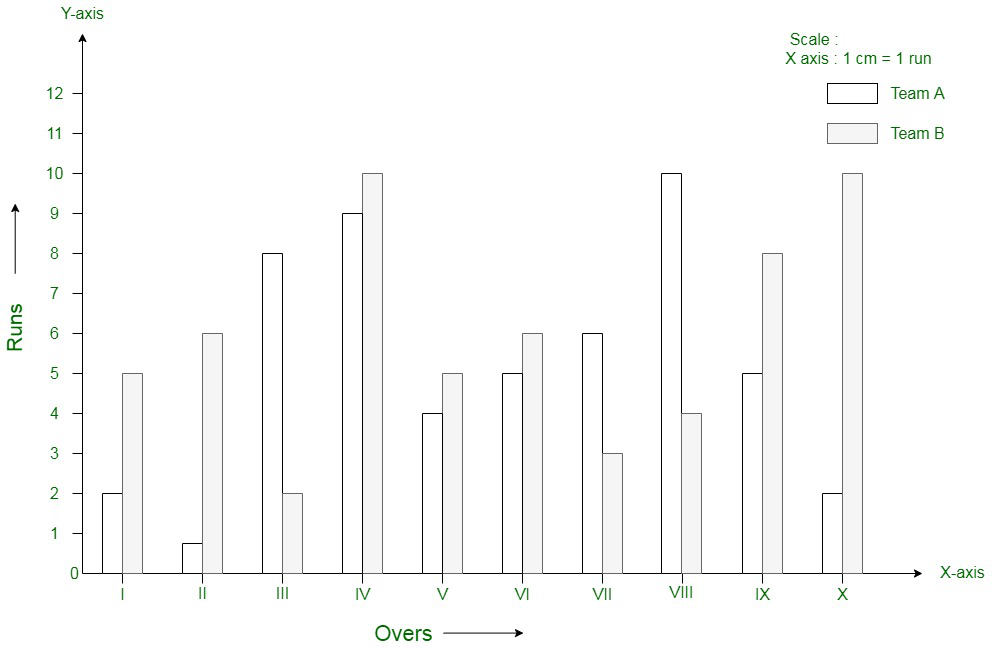My Personal Notes arrow_drop_up